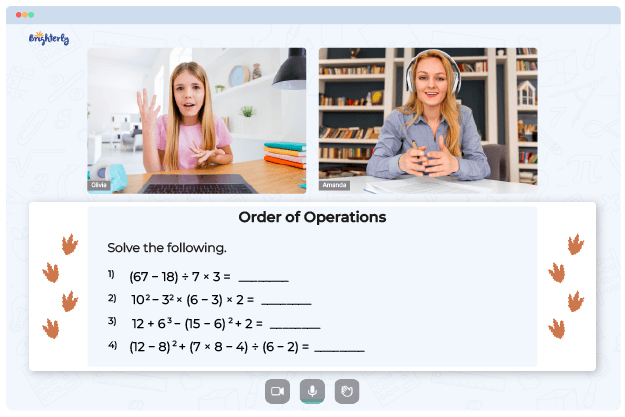# Order Of Operations Worksheets 5th Grade

Dealing with mathematics can sometimes be challenging, especially when solving problems with numerous operations. For instance, 15 – 2 x 6 equals 3, not 78 as most people may think. The order of operations can often get confusing, but what if you had a helpful guide? That is where an order of operations worksheet for 5th grade kids comes in to help students memorize how to solve math problems with ease.

## Why Do Brighterly Tutors Use Order of Operations Worksheet for 5th Grade?

Brighterly is an online platform whose tutors help kids learn math with fun and excitement. The tutors understand that math is a foundational subject, so they strive to create the most effective learning plans for students. When explaining the correct order of operations in math problems, Brighterly tutors offer students to practice with an order of operations 5th grade worksheet.

A 5th grade order of operations worksheet is divided into sections which allows kids to solve  math problems conveniently. In worksheets, students get acquainted with PEMDAS (Parenthesis, Exponents, Multiplication and Division, Addition and Subtraction). This rule helps them recall the correct sequence for tackling a math problem from left to right.

Let us use the equation 6 + 2 × (7−3)² = 38 as an example. Here is a simple breakdown of how to correctly follow the order of operations:

Math for Kids

Is Your Child Struggling With Math?
1:1 Online Math Tutoring• The first step is to do all calculations inside the parenthesis (7 – 3 = 4).
• Second, use exponents (4)² = 16.
• Perform all multiplication and division operations from the left to the right side (2 x 16 = 32).
• Do addition and subtraction (6 + 32 = 38).### Order Of Operations Worksheets 5th Grade PDF

Order Of Operations Worksheet 5Th Grade### Order Of Operations Worksheets 5th Grade PDF

5Th Grade Order Of Operations Worksheet

## Learning with Order of Operations Worksheet for 5th Grade Can Be Fun

The best tutors recommend parents to search for a 5th grade order of operations worksheet in PDF format. Thus, children are able to solve math without supervision. Using a worksheet, children can start with familiar topics such as multiplication, division, addition, and subtraction before moving on to more complex concepts like exponents and parentheses. Order of operations worksheets for 5th grade students allow kids to gain a foundation for more complex concepts introduced in subsequent years.

Children who use worksheets to solve math problems understand simple and complex order of operations faster than those who don’t. With worksheets, students can learn how to answer a wide range of problems by working through the examples. Interactive activities and questions based on the sequence of operations are included in the worksheets.

### Order of Operations Worksheets

Struggling with Order of Operations?• Does your child struggle to master the concept of order of operations?
• Try lessons with an online tutor.

Is your child having trouble grasping order of operations? An online tutor could provide the necessary support.